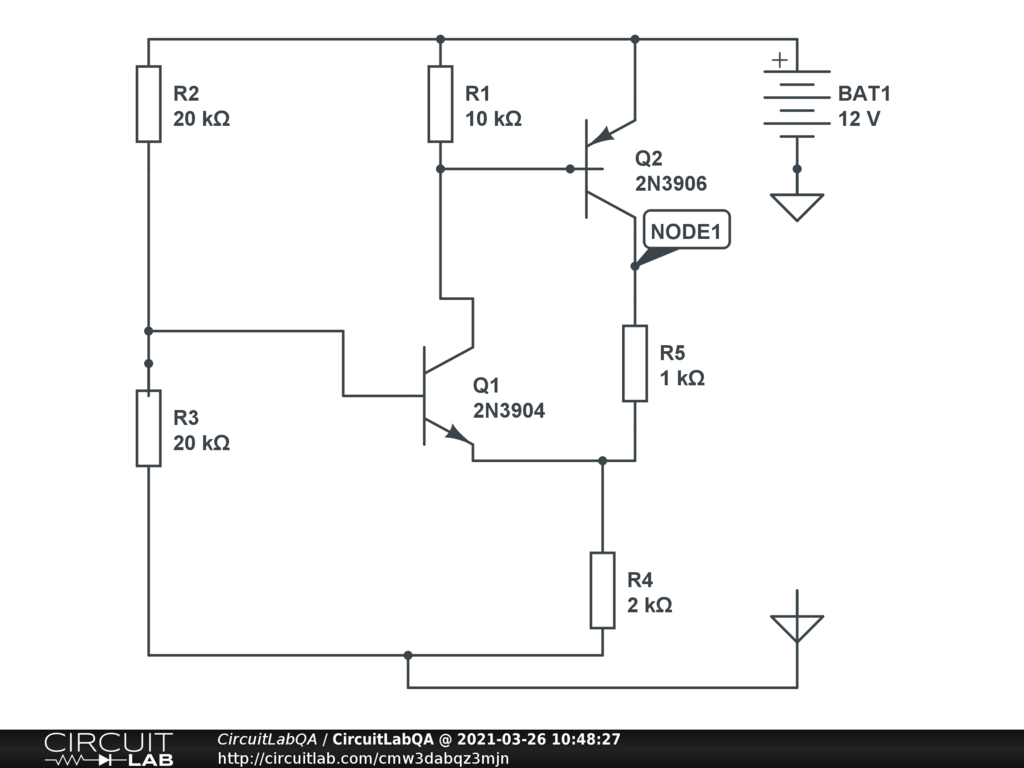## voltage divider typical biasing beta value 100 Vbe 0.7 SOLVEDfind voltage of node 1... please elaborate solution is expected by Akki7213 March 26, 2021 The simulator gives you the voltage just by mousing over the NODE1 node name. Did you already see that? by mrobbins March 26, 2021 yes. i have to solve this question with pen and paper for a test but i failed to solve that . so i needed help so posted the circuit. by Akki7213 March 26, 2021

## 1 Answer

### Answer by vanderghast

 Assuming R2 and R3 are a stiff voltage divider (to be true, the current at the base of Q1 should be small in comparison with the one through any of these two resistors), then Vr2 = Vr3 = 6 volt. Voltage loop Vr3, Vbe_q1 and Vr4: 6 = 0.7 + Vr4 (assuming Q1 is active but not blocking and its Vbe = 0.7 volt), leads to Vr4 = 5.3 volt and Ir4 =2650 uA. Since the current at the base of Q1 will then be not more than Ir4 / beta (and would be less if the current from Q2 is not zero), the stiff voltage divider assumption is considered validated. The voltage loop through Vr1 and Veb_q2 gives Vr1 =0.7 volt (assuming Q2 is active non blocking). Ir1 = 70 uA. 70 uA = Iq1_emitter ( taking Icollector = I emitter ) The sum of currents at the intersection of Q1 (its emitter), R4 and Re: Ire = ir4 - iq1_emitter = 2650 uA - 70 uA = 2580 uA Vre = ire * R2 = 2.58 volt Vnode1 = Vr4 + Vre = 5.3 volt + 2.58 volt = 7.88 volt The simulator is more precise about the Vbase_emitter values that we assumed at 0.7 volt, neither used the assumption of a stiff voltage divider. ACCEPTED 0 votes by vanderghast March 27, 2021

## Your Answer

You must log in or create an account (free!) to answer a question.

### Ask a Question

Anyone can ask a question.

Did you already search (see above) to see if a similar question has already been answered? If you can't find the answer, you may ask a question.

### About This Site

CircuitLab's Q&A site is a FREE questions and answers forum for electronics and electrical engineering students, hobbyists, and professionals.

We encourage you to use our built-in schematic & simulation software to add more detail to your questions and answers.

Acceptable Questions:

• Concept or theory questions
• Practical engineering questions
• “Homework” questions
• Software/hardware intersection
• Best practices
• Design choices & component selection
• Troubleshooting

Unacceptable Questions:

• Non-English language content
• Non-question discussion
• Non-electronics questions
• Vendor-specific topics
• Pure software questions
• CircuitLab software support

Please respect that there are both seasoned experts and total newbies here: please be nice, be constructive, and be specific!

### About CircuitLab

CircuitLab is an in-browser schematic capture and circuit simulation software tool to help you rapidly design and analyze analog and digital electronics systems.